Electron. J. Diff. Eqns., Vol. 2005(2005), No. 71, pp. 1-14.

### On Sylvester operator equations, complete trajectories, regular admissibility, and stability of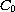-semigroups Eero Immonen

Abstract:
We show that the existence of a nontrivial bounded uniformly continuous (BUC) complete trajectory for a-semigroup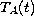generated by an operator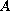in a Banach space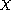is equivalent to the existence of a solution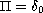to the homogenous operator equation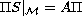. Here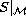generates the shift-group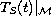in a closed translation-invariant subspace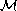of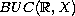, and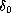is the point evaluation at the origin. If, in addition,is operator-invariant and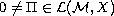is any solution of, then all functions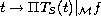,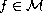, are complete trajectories forin. We connect these results to the study of regular admissibility of Banach function spaces for; among the new results are perturbation theorems for regular admissibility and complete trajectories. Finally, we show how strong stability of a-semigroup can be characterized by the nonexistence of nontrivial bounded complete trajectories for the sun-dual semigroup, and by the surjective solvability of an operator equation.

Submitted April 17, 2005. Published June 30, 2005.
Math Subject Classifications: 47D03.
Key Words: Sylvester operator equation; regularly admissible space; complete nontrivial trajectory;-semigroup; exponential stability; strong stability; exponential dichotomy.

Show me the PDF file (282K), TEX file, and other files for this article.

 Eero Immonen Institute of Mathematics Tampere University of Technology PL 553, 33101 Tampere, Finland email: Eero.Immonen@tut.fi